# Distance and Displacement Distance and Displacement Distance and

• Slides: 24Distance and Displacement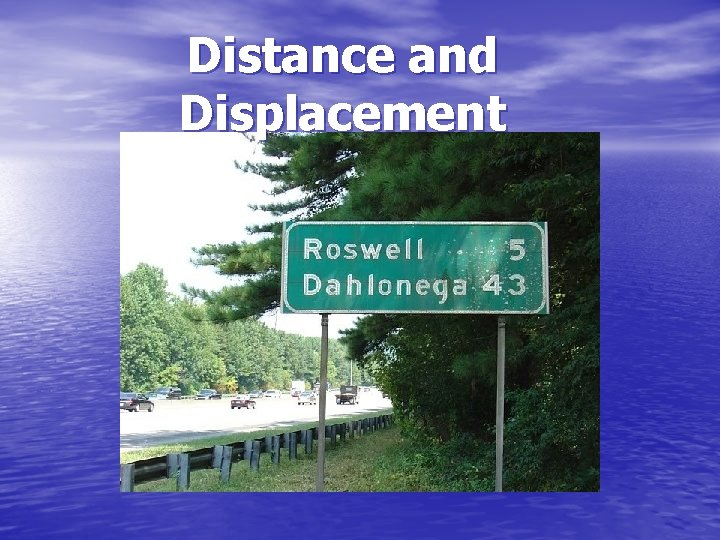Distance and Displacement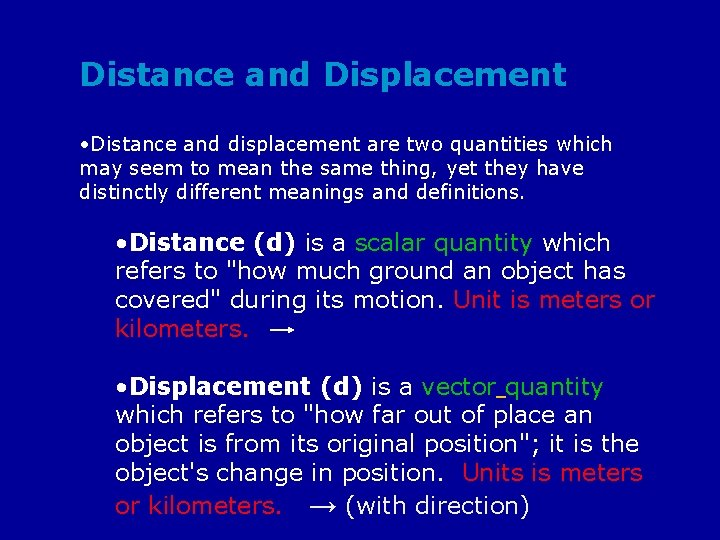Distance and Displacement • Distance and displacement are two quantities which may seem to mean the same thing, yet they have distinctly different meanings and definitions. • Distance (d) is a scalar quantity which refers to "how much ground an object has covered" during its motion. Unit is meters or kilometers. • Displacement (d) is a vector quantity which refers to "how far out of place an object is from its original position"; it is the object's change in position. Units is meters or kilometers. → (with direction)• Up or north is toward the top of your paper; can be vertical as well • Down or south is toward the bottom of your paper; can be vertical as well • Right or East or horizontal • Left or West or horizontalDelta (∆) • Greek letter used to indicate a change in a • quantity Is always calculated by: ∆ = final state – initial state Example: ∆d = positionfinal – positioninital (∆d = df – di )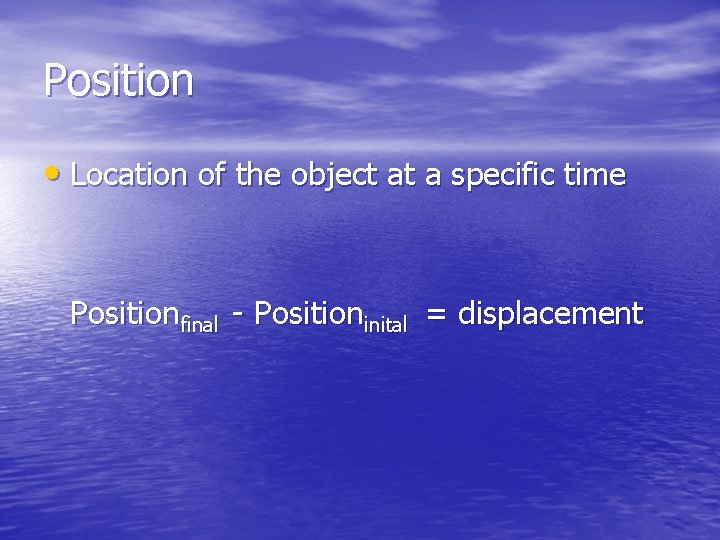Position • Location of the object at a specific time Positionfinal - Positioninital = displacementDistance vs. Displacement • You drive the path, and your odometer goes • • up by 8 miles (your distance). Your displacement is the shorter directed distance from start to stop (yellow arrow). What if you drove in a circle? start stop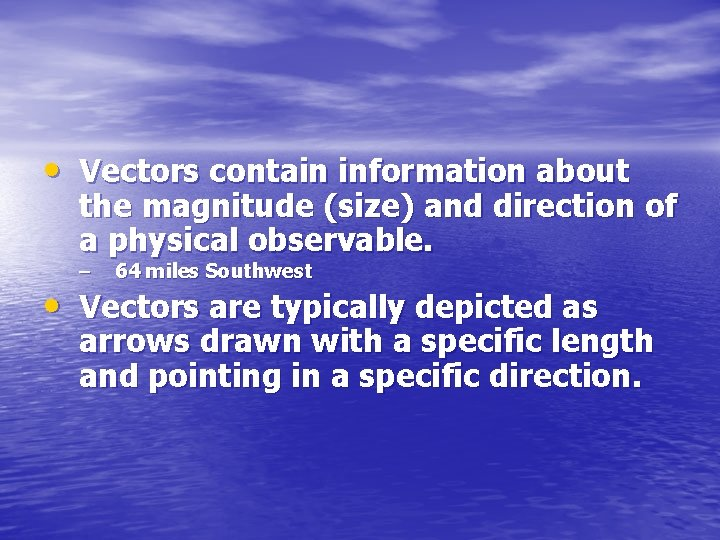• Vectors contain information about the magnitude (size) and direction of a physical observable. – 64 miles Southwest • Vectors are typically depicted as arrows drawn with a specific length and pointing in a specific direction.Some examples of vectors ….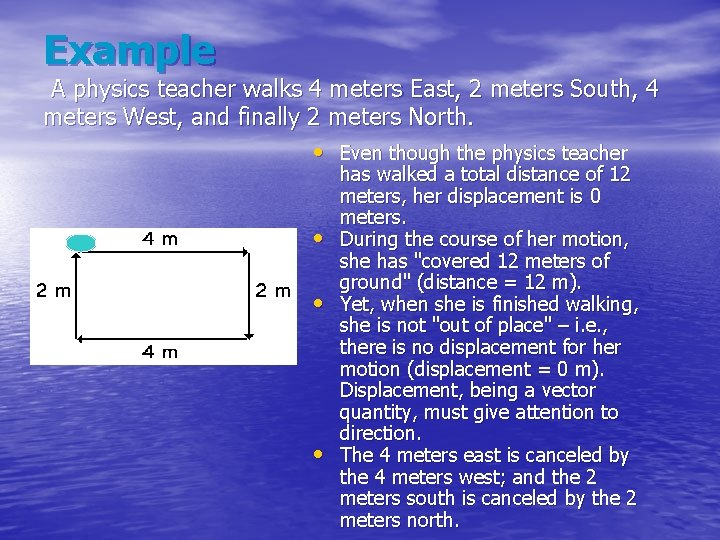Example A physics teacher walks 4 meters East, 2 meters South, 4 meters West, and finally 2 meters North. • Even though the physics teacher • • • has walked a total distance of 12 meters, her displacement is 0 meters. During the course of her motion, she has "covered 12 meters of ground" (distance = 12 m). Yet, when she is finished walking, she is not "out of place" – i. e. , there is no displacement for her motion (displacement = 0 m). Displacement, being a vector quantity, must give attention to direction. The 4 meters east is canceled by the 4 meters west; and the 2 meters south is canceled by the 2 meters north.Explanation Why does it matter? • Why does one need to understand use distance vs displacement? – Travel • Taxis: driver should operate such that distance is as close to displacement as possible…otherwise you’re being taken on the scenic (and more expensive) route • Directions: a kind local informing you that the museum you’ve been searching for is “only a few blocks from here” doesn’t help unless they include directionEvaluation Distance vs. Displacement HO 1. 5Evaluation Distance vs. Displacement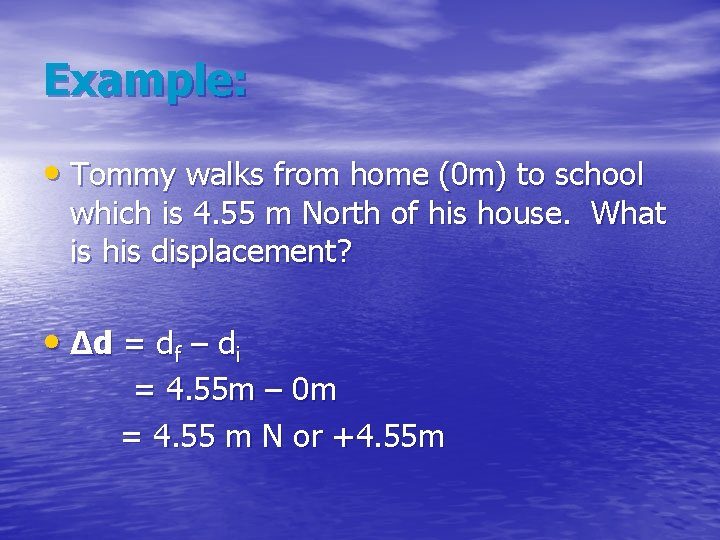Example: • Tommy walks from home (0 m) to school which is 4. 55 m North of his house. What is his displacement? • ∆d = df – di = 4. 55 m – 0 m = 4. 55 m N or +4. 55 m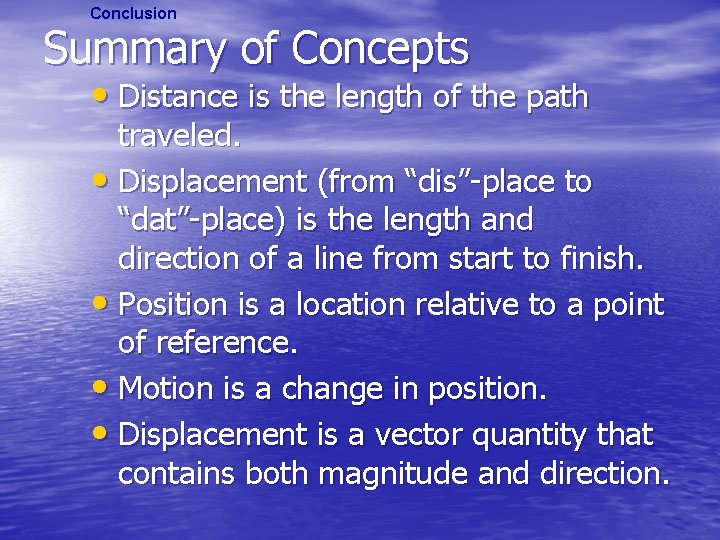Conclusion Summary of Concepts • Distance is the length of the path traveled. • Displacement (from “dis”-place to “dat”-place) is the length and direction of a line from start to finish. • Position is a location relative to a point of reference. • Motion is a change in position. • Displacement is a vector quantity that contains both magnitude and direction.Explanation "The Traveling Insectum texanum" • Distance is the actual length measured • of a particular path taken. Displacement is the length and direction of a straight line drawn from the start to finish.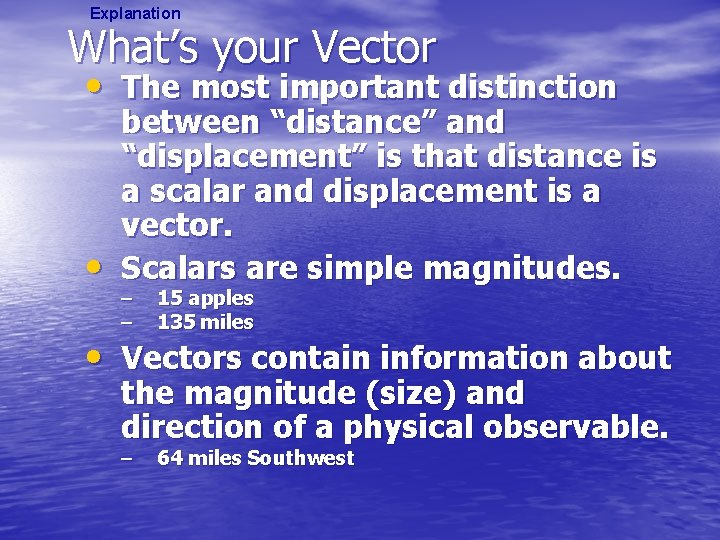Explanation What’s your Vector • The most important distinction • between “distance” and “displacement” is that distance is a scalar and displacement is a vector. Scalars are simple magnitudes. – – 15 apples 135 miles • Vectors contain information about the magnitude (size) and direction of a physical observable. – 64 miles Southwest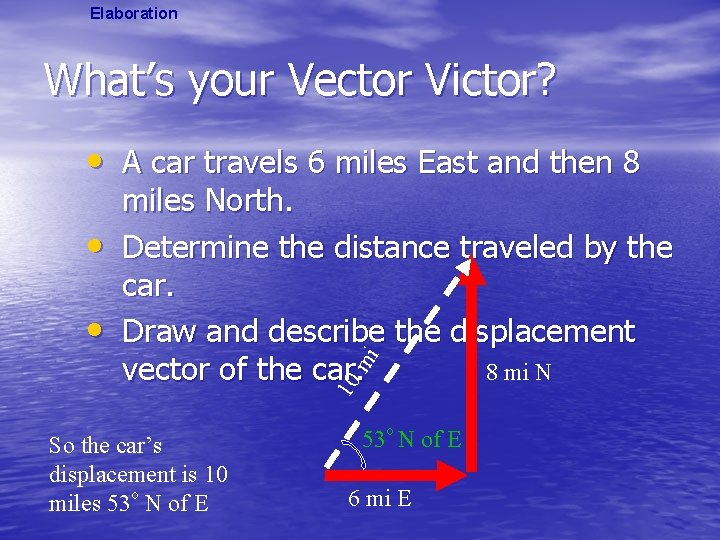Elaboration What’s your Vector Victor? • A car travels 6 miles East and then 8 • 10 mi • miles North. Determine the distance traveled by the car. Draw and describe the displacement 8 mi N vector of the car. So the car’s displacement is 10 miles 53 o N of E 6 mi E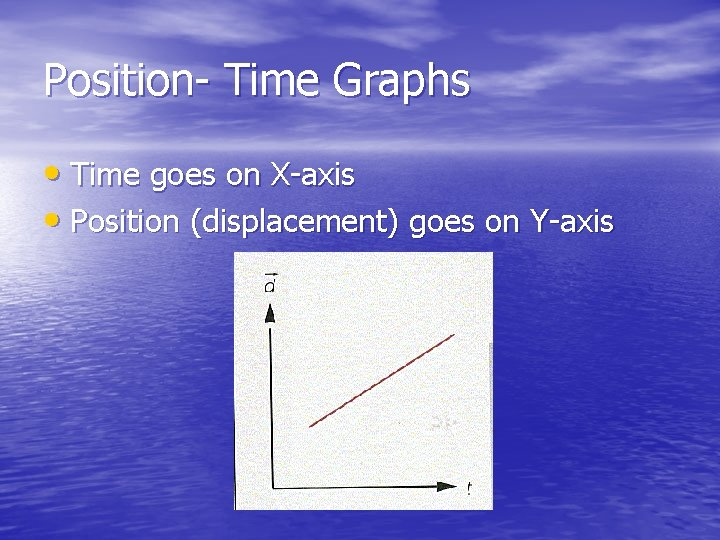Position- Time Graphs • Time goes on X-axis • Position (displacement) goes on Y-axisExploration "The Traveling Insectum texanum" 1. A bug lands on your board. This is where you will start. Label this point as “start. ” 2. What are the coordinates of this point? 3. Plot the “movement” of the Insectum texanum according to the directions below. After each move mark the arrival point and coordinates, then use that point for the next move. a. 2 units north b. 5 units west. c. 3 units north d. 3 units east e. 4 units north f. 6 units east g. Stop…. Label finish at this point.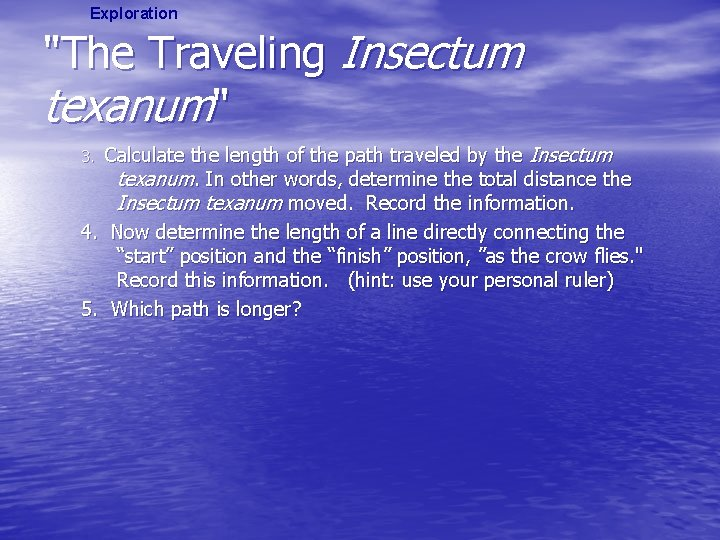Exploration "The Traveling Insectum texanum" Calculate the length of the path traveled by the Insectum texanum. In other words, determine the total distance the Insectum texanum moved. Record the information. 4. Now determine the length of a line directly connecting the “start” position and the “finish” position, ”as the crow flies. " Record this information. (hint: use your personal ruler) 5. Which path is longer? 3.Explanation "The Traveling Insectum texanum" • Physicists use the terms “distance” and “displacement” to describe • Physicists use the terms “distance” and “displacement” to describe these different measurements. • What is Distance? • What is Displacement? • Can you create a formula that will allow you to calculate: – – Distance DisplacementExample: • A dog is digging up a garden 21 m east of his house. He is scared off by a cat and ends up under a tree 6. 5 m east of his house. What is his displacement? • ∆d = df – di = 6. 5 m – 21 m = -14. 5 m (or 14. 5 m west)